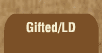Related links at Resource Room:

Mathematics and Dyslexia

Tips for Learning the Times Tables

Tools for the Times Tables & Workbook

Home > Math > times tables practice > ones times tables

## Times Tables Quiz - Stage One, Level Two

Test on Part Two, the Ones Times Tables...

Answer these questions; when you're done, click "grade me!" and you'll find out how many you got right. Use the tab key to get to the next answer, "shift - tab" to go back, or use your mouse. See how fast you can do this page!

1. 8 x 1=
2. 1 x 1=
3. 0 x 1=
4. 9 x 1=
5. 4 x 1=
6. 24 x 1=
7. 897 x 1=
8. 5 x 1=
9. 7 x 1=
10. 2 x 1=
11. 10 x 1=
12. 3 x 1=
13. 6 x 1=
14. n x 1=
15. pickle x 1=
16. Ze Bonuss! 6 x 7=

copyright © 1998-2003 Susan Jones, Resource Room. All Rights Reserved.